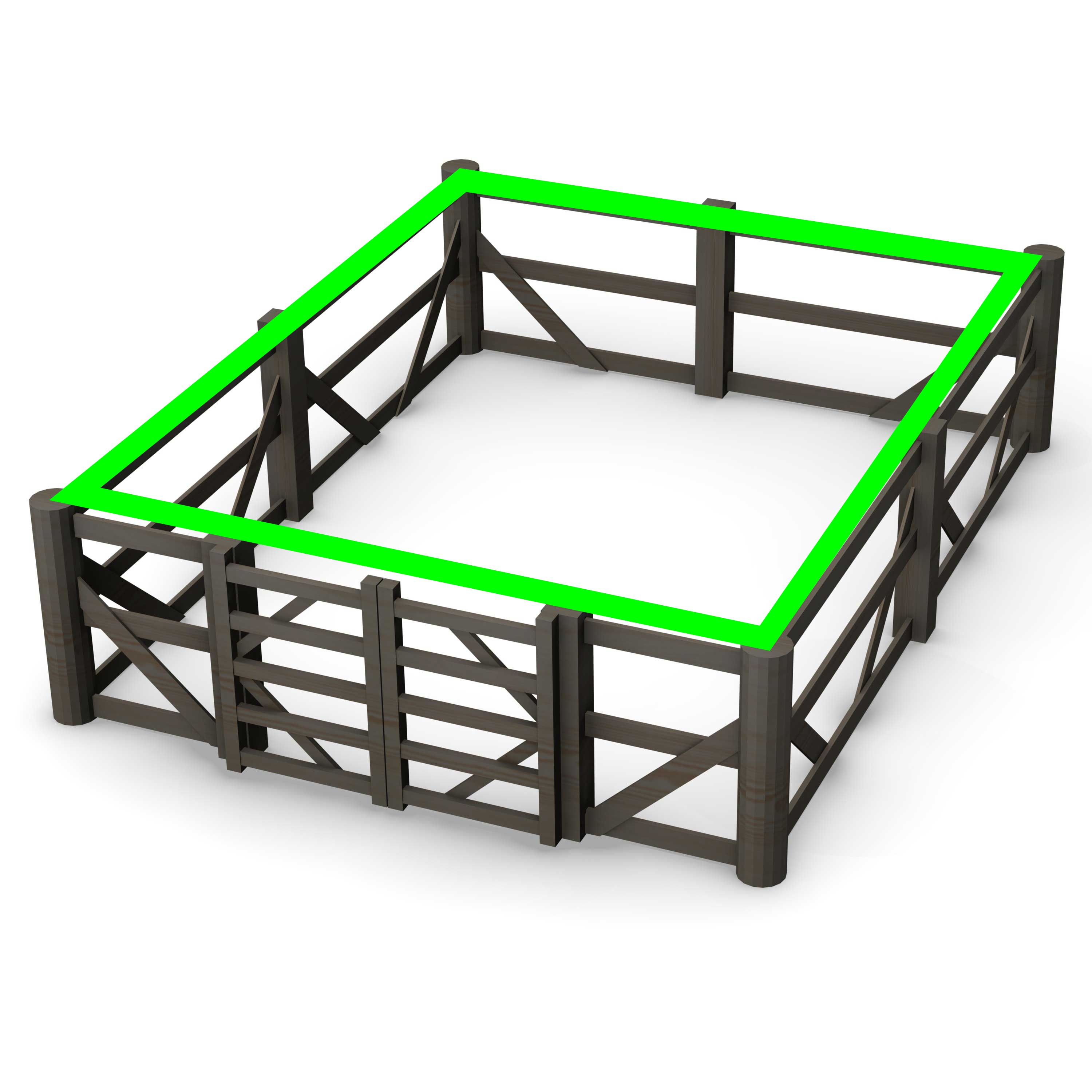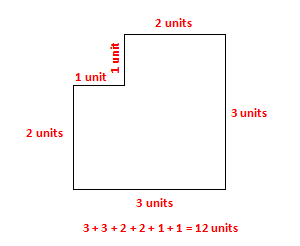# Finding the Perimeter of a Polygon

Have you ever wondered who builds all the animal pens at the zoo? How does a zoo designer know how big to make the pens? Or, how do they determine the amount of fencing needed to build a pen that has an irregular shape?The distance around a polygon is called the perimeter. To find the perimeter of any polygon you add the lengths of its sides. For example the perimeter of the irregular polygon below is 12 units.Watch the video of Ms. Ruble describing her property.

1. If her property is in the shape of a rectangle, what is the perimeter of her property?
"https://player.vimeo.com/video/64629010" webkitallowfullscreen="" width="500">

Math Perimeter from Jen Ruble on Vimeo.

# Exploring Perimeter

Explore the perimeter of different irregular polygons. Sketch three of the figures in your math journal and record the perimeter of the figure.

Perimeter Explorer

After you have correctly found the perimeter of several different irregular polygons, answer the following in your math journal:

1. What strategy did you use to determine the perimeter of the irregular polygons?
2. Which of the three figures that you sketched in your journal was the easiest to determine the perimeter? Why was it the easiest?
3. Which of the three figures that you sketched in your journal was the most difficult to determine the perimeter? Why was it the most difficult?